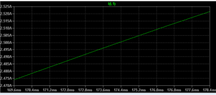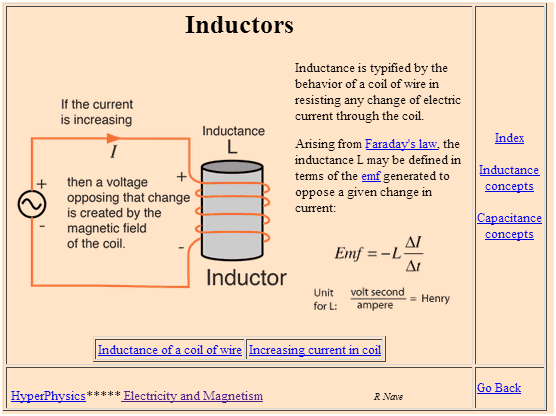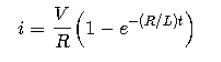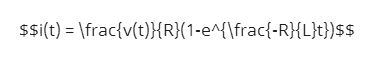# Find the time required for the current to grow to 2.5A....

IronaSona
Homework Statement:
.
Relevant Equations:
.
what is the equation on finding the ''The time required for the current to grow to ...Amps''

i was asked to find The time required for the current to grow to 2.5Amps ,and i found out that its some where around 174.38ms, using Ltspice, but i want to find it out by calculating it out.

Homework Helper
Gold Member
According to our rules, to get homework help, you need to post an attempt at a solution. Please state the problem, post any relevant diagrams and show your attempt.

IronaSona
According to our rules, to get homework help, you need to post an attempt at a solution. Please state the problem, post any relevant diagrams and show your attempt.
Q)A coil having a resistance of 33Ω and an inductance of 3.3H is connected across a 100V d.c. supply. Plot a graph of the current as a function of time using LTspice.

a)The time required for the current to grow to 2.5A

i found that its around 174ms , but i also need to find it by calculating it ,but i don't know how .

#### Attachments

•Capture.PNG
7 KB · Views: 55
Homework Helper
Gold Member
There are two ways depending on how well you wish to understand this material.
The instructive way is to write down Kirchhoff's voltage rule for the circuit, derive a differential equation and solve it on your own.
The not at all instructive way is to look up the derivation and solution on the web (keyword "RL circuit") and apply it.

IronaSona
There are two ways depending on how well you wish to understand this material.
The instructive way is to write down Kirchhoff's voltage rule for the circuit, derive a differential equation and solve it on your own.
The not at all instructive way is to look up the derivation and solution on the web (keyword "RL circuit") and apply it.
i also forgot to add , i have attempted to try it and i did 3.03(1-e^33/3.3) is that any where close ? or is this completely different equation just wanted to check

Mentor
i also forgot to add , i have attempted to try it and i did 3.03(1-e^33/3.3) is that any where close ? or is this completely different equation just wanted to check
What is the symbolic equation that you are using to come up with that number? Have you learned the differential equation for the Voltage and Current relationship for an inductor?

IronaSona
What is the symbolic equation that you are using to come up with that number? Have you learned the differential equation for the Voltage and Current relationship for an inductor?
i=v/r(1-e^-(R/L)t) ,and i haven't learn the differential equation for the Voltage and Current yet

Mentor
i=v/r(1-e^-(R/L)t)
That equation doesn't look right, but it's hard to tell with it not written in LaTeX (see the LaTeX Guide link at the lower left of the Edit window). And what is the diffference between r and R in that equation?

The equation relating voltage and current in an inductor is:

$$v(t) = L\frac{di(t)}{dt}$$http://hyperphysics.phy-astr.gsu.edu/hbase/electric/induct.html

IronaSona
That equation doesn't look right, but it's hard to tell with it not written in LaTeX (see the LaTeX Guide link at the lower left of the Edit window). And what is the diffference between r and R in that equation?

The equation relating voltage and current in an inductor is:

$$v(t) = L\frac{di(t)}{dt}$$

View attachment 281873
http://hyperphysics.phy-astr.gsu.edu/hbase/electric/induct.html
so basically that's the formula I've used ,and when you say what is the difference between r and R in that equation what do you mean by that , sorry am kind of new to all the physics part ,

http://hyperphysics.phy-astr.gsu.edu/hbase/electric/induct.html

#### Attachments

MentorAh, that's better. You were missing some parenthesis in your previous typed equation, and had mixed in r and R.

So that's a rising exponential plot, right? It starts with i(t) = 0 at t = 0, and rises quickly at first and more slowly and slowly as it approaches its final value of what?

BTW, in LaTeX it looks like this:

$$i(t) = \frac{v(t)}{R}(1-e^{\frac{-R}{L}t})$$

Which I did with this LaTeX code:Mentor
Now can you generate a more correct plot and also solve the equation for the time to 2.5A to finish the problem?IronaSona
and rises quickly at first and more slowly and slowly as it approaches its final value of what?
Its final value would be 3.03A because 100V/33R

#### Attachments

Last edited by a moderator:
•berkeman
Mentor
That looks a lot better! Can you use the simulator (does it have markers you can move along the waveform?) to answer the question about the time to 2.5A? And can you solve the exponential equation to verify that same time?Mentor
That looks a lot better! Can you use the simulator (does it have markers you can move along the waveform?) to answer the question about the time to 2.5A? And can you solve the exponential equation to verify that same time?You get extra credit from PF if you show your work in LaTeX to get t at i(t) = 2.5A.•IronaSona and SammyS